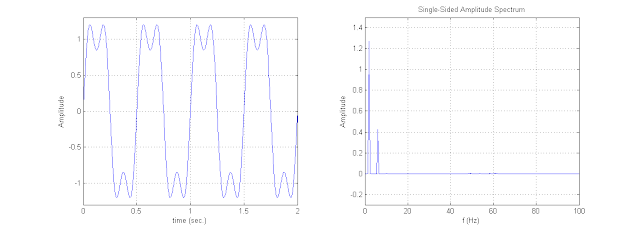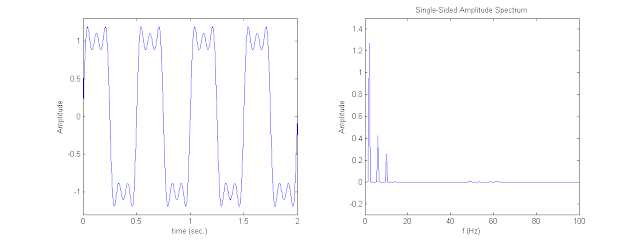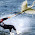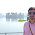### Bandwidth, Spectrum and Data rate

Let's assume a sine wave given below:

s = (4/pi)*sin(w); w = 4*pi*tThe time period of the signal is 0.5 sec (left plot) and frequency is 2 Hz (right plot). Let's assume that 2 bits are transferred by this signal in one time period.  Bit '1' is transferred by positive amplitude and bit '0' is transferred by negative amplitude.
So in 1 secs total number of bits transferred by this signal are 4.

Now let's take a signal with 2 frequency components:

s = (4/pi)*[sin(w) + (1/3)*sin(3*w)]; w = 4*pi*tHere the number of bit transferred by this signal in 1 sec = 4 (bit rate  = 4 bits/sec)
Total frequency components in the signal = 2 (Spectrum = 2 Hz and 6 Hz)
Difference between highest and lowest frequencies = 4 Hz (Bandwidth = 4 Hz)
Fundamental frequency = 2 Hz

Now let's increase the frequency components:

s = (4/pi)*[sin(w) + (1/3)*sin(3*w) + (1/5)*sin(5*w)]; w = 4*pi*tNow the number of bit transferred by this signal in 1 sec are same as previous signal = 4 (bit rate  = 4 bits/sec)
Total frequency components in the signal = 3 (Spectrum = 2 Hz, 6 Hz and 10 Hz)
Difference between highest and lowest frequencies = 8 Hz (Bandwidth = 8 Hz)
Fundamental frequency = 2 Hz

If we compare the signal with the previous signal, fundamental frequency and bit rate are same. As we increased the frequency components bandwidth of the signal also increases and the signal waveform is tending to square waveform.

Now let's increase the fundamental frequency keeping the frequency components same:

s = (4/pi)*[sin(w) + (1/3)*sin(3*w) + (1/5)*sin(5*w)]; w = 8*pi*tNow the number of bit transferred by this signal in 1 sec = 8 (bit rate  = 8 bits/sec)
Total frequency components in the signal = 3 (Spectrum = 4 Hz, 12 Hz and 20 Hz)
Difference between highest and lowest frequencies = 16 Hz (Bandwidth = 16 Hz)
Fundamental frequency = 4 Hz

If we compare above all three cases:We can increase the data rate by increasing the bandwidth and keeping the frequency components constant.
And
We can increase the accuracy by increasing the frequency components and bandwidth but data rate will be the same.

So there is a trade-off here.

Accuracy:

• Receiver must be able to interpret the received signal.

Data Rate:

• Greater the bandwidth, greater the data rate.
• Digital data is approximated by signal of limited bandwidth.

Bandwidth:

• Bandwidth is limited resource.
• Greater the bandwidth, greater the cost.
• Digital signal has infinite bandwidth, transmission signal impose limits on bandwidth of transmitted signal.

Here is a signal with 25 frequency components:

Time domain plot:

Frequency spectrum:

Now the bandwidth has increased to 192 Hz (196 Hz - 4 Hz = 192 Hz) and the bit rate is still same as 8 bits/sec but the signal has become more accurate near to square wave.
For a digital signal to transfer (for a square wave) we need infinite frequency components that means infinite sine wave and infinite bandwidth.

• Max. Data Rate calculation:

What will be the max. data rate if signals are transmitted with 8 MHz bandwidth?

For max. data rate there must not be more than 2 frequency components.
Let the fundamental frequency is  =  f
Second frequency component       = 3f

Fundamental frequency of the signal will be
3f - f  =  8 MHz
f      =  4 MHz

2 bits in one time period
2 bits in '1/f' time
2 bits in o.25 usec
8,00,000 bits in 1 sec.

So the data rate will be 8 Mb/sec

1.2.Great work Shakshama.. Keep it up!! Nice to read such blogs..

3.Well explained.

4.I have not undertood why in last case w is eguale to 6*pi*t, I expected w=8*pi*t
Thanks

1.Thanks for comment, I have changed it. It was a typing error.

5.In part Max. Data Rate calculation. why is Second frequency component = 3f ?
I sloved that.
Let the fundamental frequency is = f
Second frequency component = Af

Bandwidth of the signal will be
Af - f = 8 MHz
f = 8/(A-1) MHz
Rb=2/T=2*f=16/(A-1) =? max Rb=16 MHz when A = 2.

Did i was wrong ?

6.This comment has been removed by the author.